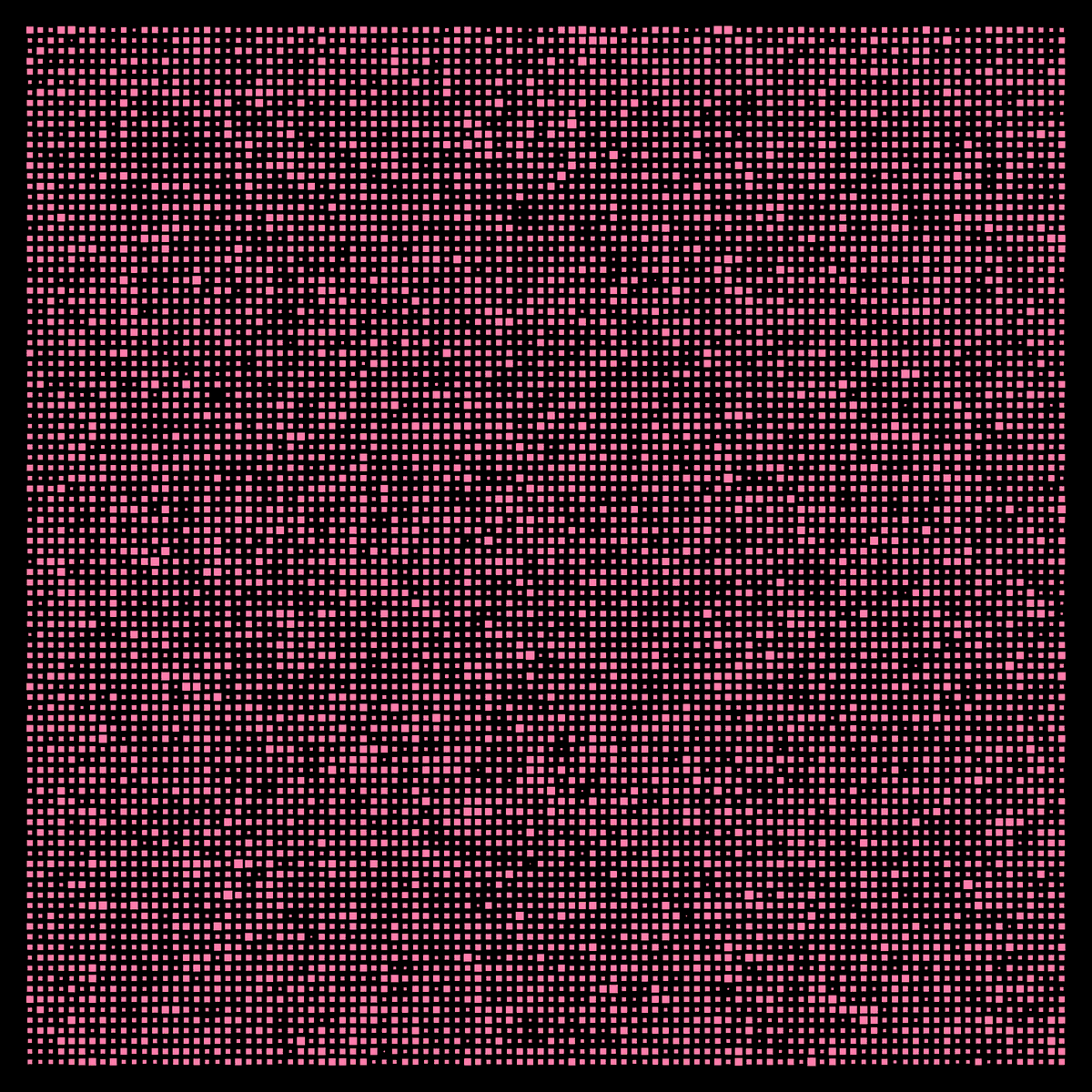I want to make a standard square hole GDS with fixed pitches in X and Y directions. 100*100 arrays.
But I want to add the random CD bias to each hole.
How to implement in Klayout?

Would be happy if anyone can share me such implementation, thanks.

• Not sure why you'd do this or how realistic you think it'll be
(much of CD variation will be systematic, and some more of
it involving proximity / edge-of-array effects). But if it were my
problem, I think I'd first make a PCell for the square object
with center 0,0 and param for size, and then a script routine
to place randomized-property cells on your major grid.

However you might as easily be able to riffle through a layout
of identical objects and property-edit the objects one by one
on their inherent geom fields.

All would seem to presume there is a random() function in
the Ruby or Python, whichever you plan to use. Seems likely.

The two seem identically easy to me because I have no chance
of accomplishing either one.

• edited February 13

Here is a python script that might do what you are looking for:

``````################################################################
#Create a grid of squares with normally distributed random size variation
#x and y dimension of squares are always equal, but could easily be adjusted to vary independantly

#main imports
import os
import pya
import numpy.random

layout = pya.Layout()

nx = 100
ny = nx
squareSize = 50.0 #microns
xpitch = 100.0 #microns
ypitch = xpitch
cdstdDev = 10.0 #microns

top = layout.create_cell("topCell")

for i in range(0,nx):
for j in range(0,ny):
thisSquareSize = squareSize + numpy.random.normal(0.0, cdstdDev)
if(thisSquareSize<0):
thisSquareSize = 0
box = pya.DBox(-thisSquareSize/2.0,-thisSquareSize/2.0,thisSquareSize/2.0,thisSquareSize/2.0).moved(i*xpitch,j*ypitch)
top.shapes(layout.layer(1, 0)).insert(box)

################################################################
#Save the file in the same directory as the script, and open it in the main window
filename = os.path.join(os.path.dirname(__file__), "squares.gds")
layout.write(filename)
################################################################
``````

Image of output gds:• Cool!Many thanks for sharing this script.

One remark: in order to be useful for Linux users, the path separator cannot be a backslash. For the generic version use:

``````...
filename = os.path.join(os.path.dirname(__file__), "squares.gds")
...
``````

Matthias

• Thanks Matthias, I have edited the above script to include that correction.